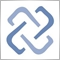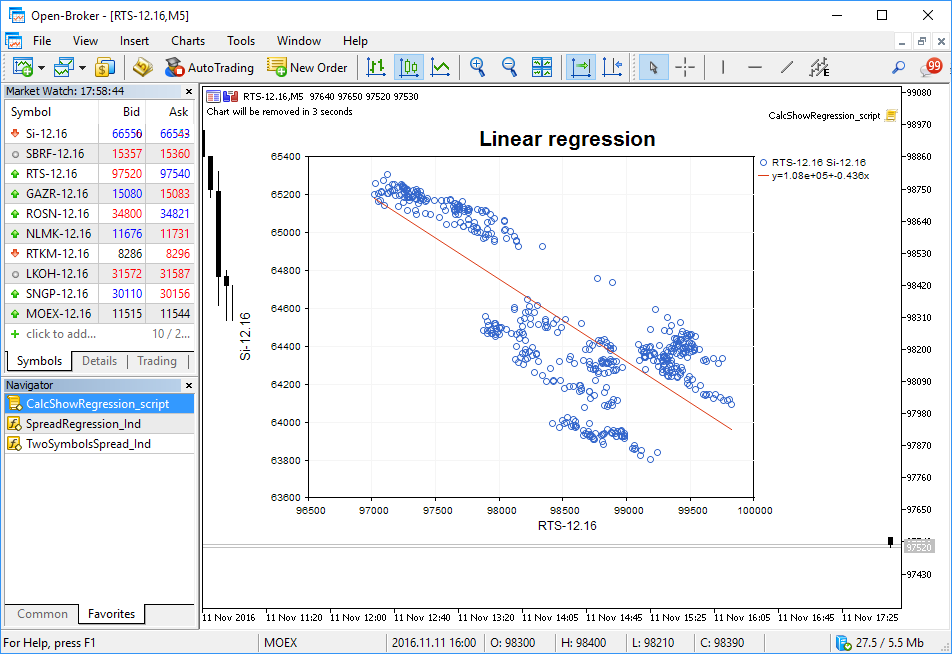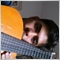# Discussion of article "An Example of Developing a Spread Strategy for Moscow Exchange Futures"Moderator
250724

New article An Example of Developing a Spread Strategy for Moscow Exchange Futures has been published:

The MetaTrader 5 platform allows developing and testing trading robots that simultaneously trade multiple financial instruments. The built-in Strategy Tester automatically downloads required tick history from the broker's server taking into account contract specifications, so the developer does not need to do anything manually. This makes it possible to easily and reliably reproduce trading environment conditions, including even millisecond intervals between the arrival of ticks on different symbols. In this article we will demonstrate the development and testing of a spread strategy on two Moscow Exchange futures.

We can express correlation between the two assets using a linear regression equation Y(X)=A(X)+B. Let's create a script CalcShowRegression_script.mq5, which takes two arrays of close prices, calculates coefficients and shows the distribution diagram with a regression line straight on the chart.

Regression coefficients are calculated using an ALGLIB function, and the values are drawn using graphic classes of the standard library.Author: MetaQuotes Software Corp.1515

m_graphic.YAxis().ValuesWith(45);  // Length of values along the Y axis error here